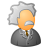# App Development

Announcements
April 22, 2PM EST: Learn about GeoOperations in Qlik Sense SaaS READ MORE
cancel
Showing results for
Did you mean:Contributor III

## Front end expression Help

Hi all ,

PFA excel . i have 3 columns - Quarter(A) , Per(B) and ISP(C) ( PFA excel )

I need sum of column ISP (C) where column B(Per) and Column (ISP ) is greater than 0.

=sum({<ISP={"=RangeSum(Per,ISP)>0"}>}ISP)

Value should be - 2764339

Labels (2)

• ### front end

1 Solution

Accepted SolutionsMVP

May be try this expression

``=Sum({<ISP = {">0"}>*<Per = {">0"}>}ISP)``

If you want to do this in script... you can create a flag in the script

``````Table:
Per,
ISP,
If(Per > 0 and ISP > 0, 1, 0) as Flag
FROM
(ooxml, embedded labels, table is Sheet1);``````

and then use this expression

``=Sum({<Flag = {'1'}>}ISP)``

3 RepliesMVP

May be try this expression

``=Sum({<ISP = {">0"}>*<Per = {">0"}>}ISP)``

If you want to do this in script... you can create a flag in the script

``````Table:
Per,
ISP,
If(Per > 0 and ISP > 0, 1, 0) as Flag
FROM
(ooxml, embedded labels, table is Sheet1);``````

and then use this expression

``=Sum({<Flag = {'1'}>}ISP)``Contributor III
Author

Thanks Sunny
its working fine for the example which i provided

But in my dashboard I'm getting zero .. any idea Y ?

I have attached the screen shot .MVP

Just by looking at the screenshots... no idea